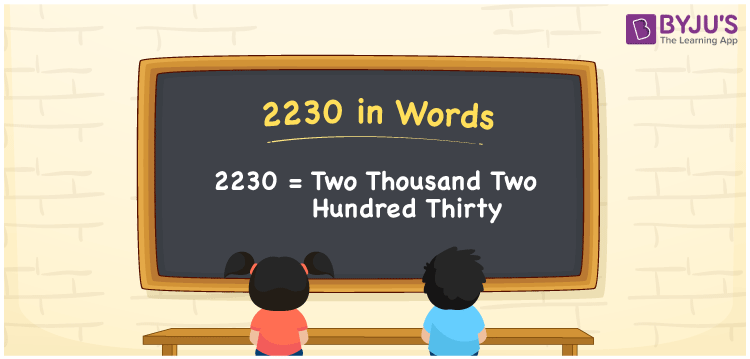# 2230 in Words

2230 in words can be written as Two Thousand Two Hundred Thirty. The number 2230 is written in words using the English alphabet. For example, if you buy earphones for Rs. 2230, then you can say that “I bought earphones for Two Thousand Two Hundred Thirty Rupees”. The numbers in words concept explained here will provide you in depth knowledge of basic concepts. Hence, 2230 can be read as “Two Thousand Two Hundred Thirty” in English.

 2230 in words Two Thousand Two Hundred Thirty Two Thousand Two Hundred Thirty in Numbers 2230

## 2230 in English Words## How to Write 2230 in Words?

Given below is the place value chart of the number 2230. The number is written in words by knowing the place value of each digit.

 Thousand Hundreds Tens Ones 2 2 3 0

The expanded form of 2230 is:

2 x Thousand + 2 × Hundred + 3 × Ten + 0 × One

= 2 x 1000 + 2 × 100 + 3 × 10 + 0 × 1

= 2000 + 200 + 30

= 2230

= Two Thousand Two Hundred Thirty

Therefore, 2230 in words is written as Two Thousand Two Hundred Thirty.

2230 is a natural number that precedes 2231 and succeeds 2229.

2230 in words – Two Thousand Two Hundred Thirty

Is 2230 an odd number? – No

Is 2230 an even number? – Yes

Is 2230 a perfect square number? – No

Is 2230 a perfect cube number? – No

Is 2230 a prime number? – No

Is 2230 a composite number? – Yes

## Frequently Asked Questions on 2230 in Words

Q1

### Write 2230 in words.

2230 can be written in words as “Two Thousand Two Hundred Thirty”.
Q2

### How do you write Two Thousand Two Hundred Thirty in numbers?

Two Thousand Two Hundred Thirty can be written in numbers as 2230.
Q3

### Is 2230 a perfect cube number?

No, 2230 is not a perfect cube number as it is not the product of three similar numbers.